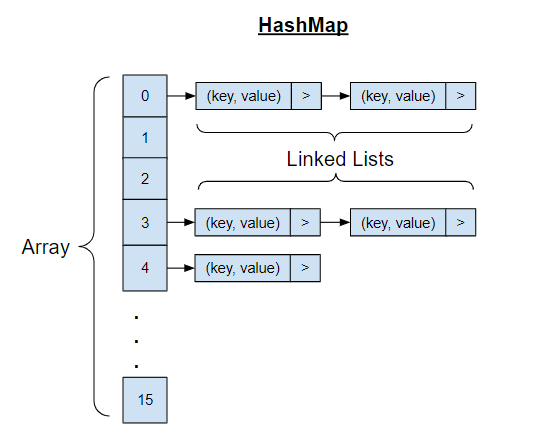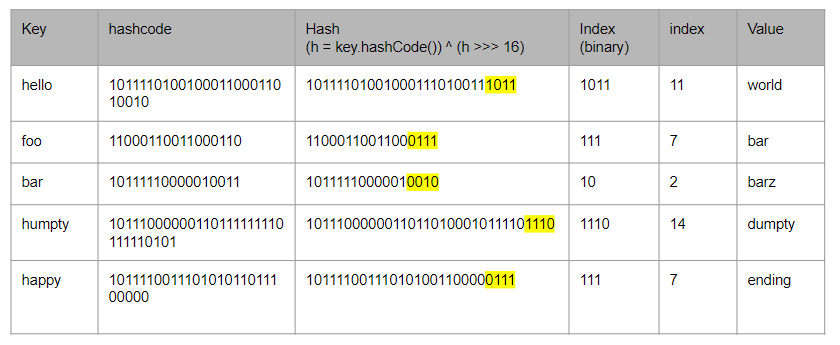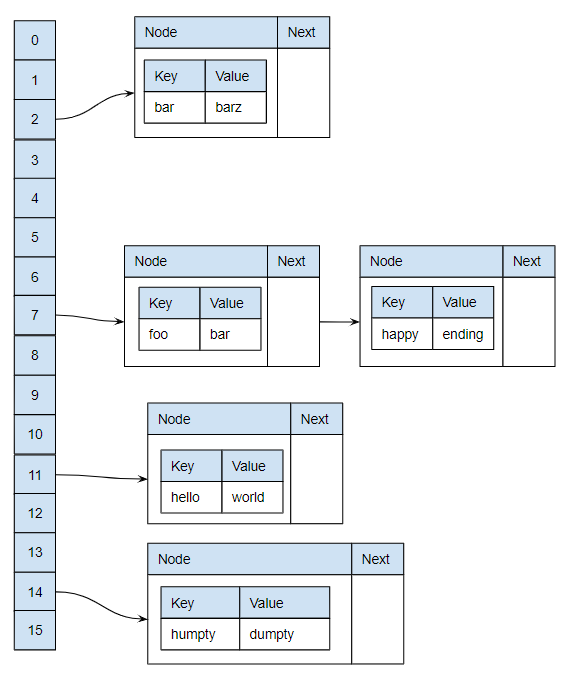# Java HashMap ExplainedThe implementation details of the java hashmap is a very common interview question for java developers, though I’m strongly against asking such question for interviews (because it is like asking how a car works in a driving test), I’m here to try to explain it as simple as I can because I can’t find the simplest accurate explanation online. This article is for someone with basic programming knowledge, able to use arrays, linked lists and hashmaps.

TLDR; It’s an array of linked list. Ideally, each item in the array is a linked list of just 1 node. So you can get `O(1)` complexity to perform `get` and `put` by calculating the `index` of the array by hashing the `key` of the hashmap, and get the single item in the linked list. However, because hash function doesn’t have to create a unique hash code for different values, when more than 1 key have the same hash code, they are added to the linked list. So, to get to the correct key, you’ll need to traverse the linked list and compare the key one by one, thus resulting in a complexity of `O(n)` for those keys with the same hash code.

To understand how hashmap works, we first need to understand the goal we want to achieve. Then we make use of what is available to achieve the goal.

Goal: To store and retrieve a list of data by using a non numeric key with the least complexity possible.

What is available: Arrays, Linked Lists, Sets, Trees

A naive approach is to store the key value pair in any of the data structures above, and iterate and compare the keys in the nodes one by one. But that is not efficient, resulting in a complexity of O(n). The least complexity possible is O(1), achievable by using arrays. We just need to know the exact index in the array. However, since we wanted a non numeric key for our hashmap, that is not so straightforward.

We can achieve that by turning the key into an integer. How? hashCode(). Every java object has a hashcode() function, to hash the object into an integer. But do take note of the contract.

1. The hashcode function must return the same value for the same unmodified object in an application. Though the same object do not need to return the same value when run in a different application.

2. If 2 objects return true with the `equals()` function, they must have the same hashCode value.

3. Different objects can return the same hashcode even if they are not equal.

So with the hashCode function, we can make an integer from keys of any type. However the integer is going to be very big, like 99162322. We can’t just create an array with the size of the largest integer allowed and use only a few slots. In fact, by default, the java hashmaps are created with only a size 16 array. But it has a built in mechanism to double the size of the array whenever the array is 75% filled. So a simple `bitwise AND operation` with the size of the current array is performed on the hash to determine the index.

For example, suppose you have a key `hello`, the hash is `99162322`, the bit value will be `101111010010001100011010010`, and the size of the current array is just 16. So we’ll do a bitwise AND operation `101111010010001100011010010 & 1111`, which gives us `0010`, which is 2. So we’ll allocate the key `hello` to index 2 of the array.

Note that we `bitwise AND` 15 which is `1111`, instead of 16 because programmers start counting from 0, so the 16th index is actually 15.

Tada! But then don’t you think different keys can have the same hashCode since we are just taking the last few bytes? Yes, but that is alright, because each item in the array is a linked list. So when that happens, we just add it to the linked list. And in each node of the linked list, we store both the `key` and the `value` of the hashmap entry. So if there are more than 1 node in the linked list, we’ll need to iterate through the linked list and compare the `key` one by one.

This above is the general idea of how a hashmap functions, there are quite a number of tweaks to make it more efficient, for example:

1. The hashmap doesn’t use the hash of the key directly as the index, instead it applies another hash function `(key == null) ? 0 : (h = key.hashCode()) ^ (h >>> 16)` to the key before the bitwise AND operation to make it less likely to collide (multiple keys having the same index).

2. When there are more than 8 nodes in a linked list, it will transform to use a tree instead.

But the information above should be enough, afterall, writing software is about reusing what is available, you shouldn’t need to know how all your node dependencies work in order to use them.

To further illustrate the point, suppose a hashmap with the following key value pairs, the hashCode, hash and index generated from the key are shown in the table below.The result hashmap in the array and linked list combination will be as such.The code to generate the keys are available on my github gist

``````class HashMapDemo {
public static void main(String[ ] args) {
String key = "superman";
int size = 15;
System.out.println("Key: " + key);
int hashCode = key.hashCode();
System.out.println("HashCode: " + hashCode);
System.out.println("HashCode (Binary): " + Integer.toBinaryString(hashCode));
int h;
int hash = (h = key.hashCode()) ^ (h >>> 16);
System.out.println("Hash: " + hash);
System.out.println("Hash (Binary): " + Integer.toBinaryString(hash));
System.out.println("Size (Binary): " + Integer.toBinaryString(size));
int x = size & hash;
System.out.println("Location: " + x);
System.out.println(Integer.toBinaryString(x));

// Key: superman
// HashCode: -1673281537
// HashCode (Binary): 10011100010000111011111111111111
// Hash: -1673321540
// Hash (Binary): 10011100010000110010001110111100
// Size (Binary): 1111
// Location: 12
// 1100
}
}
``````

Tags:

Categories:

Updated: